# Group (mathematics)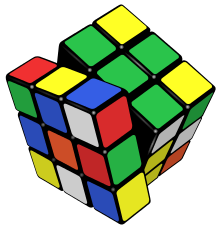The manipulations of this Rubik's Cube form the Rubik's Cube group.

In mathematics, a group is an algebraic structure consisting of a set of elements equipped with an operation that combines any two elements to form a third element. The operation satisfies four conditions called the group axioms, namely closure, associativity, identity and invertibility. One of the most familiar examples of a group is the set of integers together with the addition operation, but the abstract formalization of the group axioms, detached as it is from the concrete nature of any particular group and its operation, applies much more widely. It allows entities with highly diverse mathematical origins in abstract algebra and beyond to be handled in a flexible way while retaining their essential structural aspects. The ubiquity of groups in numerous areas within and outside mathematics makes them a central organizing principle of contemporary mathematics.

Groups share a fundamental kinship with the notion of symmetry. For example, a symmetry group encodes symmetry features of a geometrical object: the group consists of the set of transformations that leave the object unchanged and the operation of combining two such transformations by performing one after the other. Lie groups are the symmetry groups used in the Standard Model of particle physics; Poincaré groups, which are also Lie groups, can express the physical symmetry underlying special relativity; and point groups are used to help understand symmetry phenomena in molecular chemistry.

The concept of a group arose from the study of polynomial equations, starting with Évariste Galois in the 1830s. After contributions from other fields such as number theory and geometry, the group notion was generalized and firmly established around 1870. Modern group theory—an active mathematical discipline—studies groups in their own right.a[] To explore groups, mathematicians have devised various notions to break groups into smaller, better-understandable pieces, such as subgroups, quotient groups and simple groups. In addition to their abstract properties, group theorists also study the different ways in which a group can be expressed concretely, both from a point of view of representation theory (that is, through the representations of the group) and of computational group theory. A theory has been developed for finite groups, which culminated with the classification of finite simple groups, completed in 2004.aa[] Since the mid-1980s, geometric group theory, which studies finitely generated groups as geometric objects, has become a particularly active area in group theory.

## Definition and illustration

### First example: the integers

One of the most familiar groups is the set of integers Z which consists of the numbers

..., −4, −3, −2, −1, 0, 1, 2, 3, 4, ..., together with addition.

The following properties of integer addition serve as a model for the abstract group axioms given in the definition below.

• For any two integers a and b, the sum a + b is also an integer. That is, addition of integers always yields an integer. This property is known as closure under addition.
• For all integers a, b and c, (a + b) + c = a + (b + c). Expressed in words, adding a to b first, and then adding the result to c gives the same final result as adding a to the sum of b and c, a property known as associativity.
• If a is any integer, then 0 + a = a + 0 = a. Zero is called the identity element of addition because adding it to any integer returns the same integer.
• For every integer a, there is an integer b such that a + b = b + a = 0. The integer b is called the inverse element of the integer a and is denoted −a.

The integers, together with the operation +, form a mathematical object belonging to a broad class sharing similar structural aspects. To appropriately understand these structures as a collective, the following abstract definition is developed.

### Definition

[T]he axioms for a group are short and natural... Yet somehow hidden behind these axioms is the monster simple group, a huge and extraordinary mathematical object, which appears to rely on numerous bizarre coincidences to exist. The axioms for groups give no obvious hint that anything like this exists.

Richard Borcherds in Mathematicians: An Outer View of the Inner World 

A group is a set, G, together with an operation • (called the group law of G) that combines any two elements a and b to form another element, denoted ab or ab. To qualify as a group, the set and operation, (G, •), must satisfy four requirements known as the group axioms:

Closure
For all a, b in G, the result of the operation, ab, is also in G.b[]
Associativity
For all a, b and c in G, (ab) • c = a • (bc).
Identity element
There exists an element e in G, such that for every element a in G, the equation ea = ae = a holds. Such an element is unique (see below), and thus one speaks of the identity element.
Inverse element
For each a in G, there exists an element b in G, commonly denoted a−1 (or −a, if the operation is denoted "+"), such that ab = ba = e, where e is the identity element.

The result of an operation may depend on the order of the operands. In other words, the result of combining element a with element b need not yield the same result as combining element b with element a; the equation

ab = ba

may not always be true. This equation always holds in the group of integers under addition, because a + b = b + a for any two integers (commutativity of addition). Groups for which the commutativity equation ab = ba always holds are called abelian groups (in honor of Niels Henrik Abel). The symmetry group described in the following section is an example of a group that is not abelian.

The identity element of a group G is often written as 1 or 1G, a notation inherited from the multiplicative identity. If a group is abelian, then one may choose to denote the group operation by + and the identity element by 0; in that case, the group is called an additive group. The identity element can also be written as id.

The set G is called the underlying set of the group (G, •). Often the group's underlying set G is used as a short name for the group (G, •). Along the same lines, shorthand expressions such as "a subset of the group G" or "an element of group G" are used when what is actually meant is "a subset of the underlying set G of the group (G, •)" or "an element of the underlying set G of the group (G, •)". Usually, it is clear from the context whether a symbol like G refers to a group or to an underlying set.

### Second example: a symmetry group

Two figures in the plane are congruent if one can be changed into the other using a combination of rotations, reflections, and translations. Any figure is congruent to itself. However, some figures are congruent to themselves in more than one way, and these extra congruences are called symmetries. A square has eight symmetries. These are: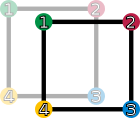id (keeping it as it is)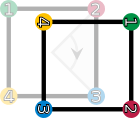r1 (rotation by 90° clockwise)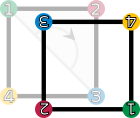r2 (rotation by 180° clockwise)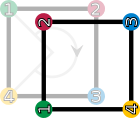r3 (rotation by 270° clockwise)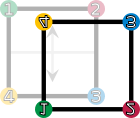fv (vertical reflection)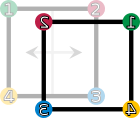fh (horizontal reflection)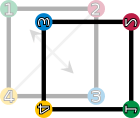fd (diagonal reflection)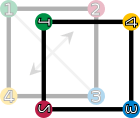fc (counter-diagonal reflection)
• the identity operation leaving everything unchanged, denoted id;
• rotations of the square around its center by 90° clockwise, 180° clockwise, and 270° clockwise, denoted by r1, r2 and r3, respectively;
• reflections about the vertical and horizontal middle line (fh and fv), or through the two diagonals (fd and fc).

These symmetries are represented by functions. Each of these functions sends a point in the square to the corresponding point under the symmetry. For example, r1 sends a point to its rotation 90° clockwise around the square's center, and fh sends a point to its reflection across the square's vertical middle line. Composing two of these symmetry functions gives another symmetry function. These symmetries determine a group called the dihedral group of degree 4 and denoted D4. The underlying set of the group is the above set of symmetry functions, and the group operation is function composition. Two symmetries are combined by composing them as functions, that is, applying the first one to the square, and the second one to the result of the first application. The result of performing first a and then b is written symbolically from right to left as

ba ("apply the symmetry b after performing the symmetry a").

The right-to-left notation is the same notation that is used for composition of functions.

The group table on the right lists the results of all such compositions possible. For example, rotating by 270° clockwise (r3) and then reflecting horizontally (fh) is the same as performing a reflection along the diagonal (fd). Using the above symbols, highlighted in blue in the group table:

fh • r3 = fd.
Group table of D4
id r1 r2 r3 fv fh fd fc
id id r1 r2 r3 fv fh fd fc
r1 r1 r2 r3 id fc fd fv fh
r2 r2 r3 id r1 fh fv fc fd
r3 r3 id r1 r2 fd fc fh fv
fv fv fd fh fc id r2 r1 r3
fh fh fc fv fd r2 id r3 r1
fd fd fh fc fv r3 r1 id r2
fc fc fv fd fh r1 r3 r2 id
The elements id, r1, r2, and r3 form a subgroup, highlighted in      red (upper left region). A left and right coset of this subgroup is highlighted in      green (in the last row) and      yellow (last column), respectively.

Given this set of symmetries and the described operation, the group axioms can be understood as follows:

1. The closure axiom demands that the composition ba of any two symmetries a and b is also a symmetry. Another example for the group operation is
r3 • fh = fc,
i.e. rotating 270° clockwise after reflecting horizontally equals reflecting along the counter-diagonal (fc). Indeed every other combination of two symmetries still gives a symmetry, as can be checked using the group table.
2. The associativity constraint deals with composing more than two symmetries: Starting with three elements a, b and c of D4, there are two possible ways of using these three symmetries in this order to determine a symmetry of the square. One of these ways is to first compose a and b into a single symmetry, then to compose that symmetry with c. The other way is to first compose b and c, then to compose the resulting symmetry with a. The associativity condition
(ab) • c = a • (bc)

means that these two ways are the same, i.e., a product of many group elements can be simplified in any grouping. For example, (fd • fv) • r2 = fd • (fv • r2) can be checked using the group table at the right

 (fd • fv) • r2 = r3 • r2 = r1, which equals fd • (fv • r2) = fd • fh = r1.
While associativity is true for the symmetries of the square and addition of numbers, it is not true for all operations. For instance, subtraction of numbers is not associative: (7 − 3) − 2 = 2 is not the same as 7 − (3 − 2) = 6.
3. The identity element is the symmetry id leaving everything unchanged: for any symmetry a, performing id after a (or a after id) equals a, in symbolic form,
id • a = a,
a • id = a.
4. An inverse element undoes the transformation of some other element. Every symmetry can be undone: each of the following transformations—identity id, the reflections fh, fv, fd, fc and the 180° rotation r2—is its own inverse, because performing it twice brings the square back to its original orientation. The rotations r3 and r1 are each other's inverses, because rotating 90° and then rotation 270° (or vice versa) yields a rotation over 360° which leaves the square unchanged. In symbols,
fh • fh = id,
r3 • r1 = r1 • r3 = id.

In contrast to the group of integers above, where the order of the operation is irrelevant, it does matter in D4: fh • r1 = fc but r1 • fh = fd. In other words, D4 is not abelian, which makes the group structure more difficult than the integers introduced first.

## History

The modern concept of an abstract group developed out of several fields of mathematics. The original motivation for group theory was the quest for solutions of polynomial equations of degree higher than 4. The 19th-century French mathematician Évariste Galois, extending prior work of Paolo Ruffini and Joseph-Louis Lagrange, gave a criterion for the solvability of a particular polynomial equation in terms of the symmetry group of its roots (solutions). The elements of such a Galois group correspond to certain permutations of the roots. At first, Galois' ideas were rejected by his contemporaries, and published only posthumously. More general permutation groups were investigated in particular by Augustin Louis Cauchy. Arthur Cayley's On the theory of groups, as depending on the symbolic equation θn = 1 (1854) gives the first abstract definition of a finite group.

Geometry was a second field in which groups were used systematically, especially symmetry groups as part of Felix Klein's 1872 Erlangen program. After novel geometries such as hyperbolic and projective geometry had emerged, Klein used group theory to organize them in a more coherent way. Further advancing these ideas, Sophus Lie founded the study of Lie groups in 1884.

The third field contributing to group theory was number theory. Certain abelian group structures had been used implicitly in Carl Friedrich Gauss' number-theoretical work Disquisitiones Arithmeticae (1798), and more explicitly by Leopold Kronecker. In 1847, Ernst Kummer made early attempts to prove Fermat's Last Theorem by developing groups describing factorization into prime numbers.

The convergence of these various sources into a uniform theory of groups started with Camille Jordan's Traité des substitutions et des équations algébriques (1870). Walther von Dyck (1882) introduced the idea of specifying a group by means of generators and relations, and was also the first to give an axiomatic definition of an "abstract group", in the terminology of the time. As of the 20th century, groups gained wide recognition by the pioneering work of Ferdinand Georg Frobenius and William Burnside, who worked on representation theory of finite groups, Richard Brauer's modular representation theory and Issai Schur's papers. The theory of Lie groups, and more generally locally compact groups was studied by Hermann Weyl, Élie Cartan and many others. Its algebraic counterpart, the theory of algebraic groups, was first shaped by Claude Chevalley (from the late 1930s) and later by the work of Armand Borel and Jacques Tits.

The University of Chicago's 1960–61 Group Theory Year brought together group theorists such as Daniel Gorenstein, John G. Thompson and Walter Feit, laying the foundation of a collaboration that, with input from numerous other mathematicians, led to the classification of finite simple groups, with the final step taken by Aschbacher and Smith in 2004. This project exceeded previous mathematical endeavours by its sheer size, in both length of proof and number of researchers. Research is ongoing to simplify the proof of this classification. These days, group theory is still a highly active mathematical branch, impacting many other fields.a[]

## Elementary consequences of the group axioms

Basic facts about all groups that can be obtained directly from the group axioms are commonly subsumed under elementary group theory. For example, repeated applications of the associativity axiom show that the unambiguity of

abc = (ab) • c = a • (bc)

generalizes to more than three factors. Because this implies that parentheses can be inserted anywhere within such a series of terms, parentheses are usually omitted.

The axioms may be weakened to assert only the existence of a left identity and left inverses. Both can be shown to be actually two-sided, so the resulting definition is equivalent to the one given above.

### Uniqueness of identity element and inverses

Two important consequences of the group axioms are the uniqueness of the identity element and the uniqueness of inverse elements. There can be only one identity element in a group, and each element in a group has exactly one inverse element. Thus, it is customary to speak of the identity, and the inverse of an element.

To prove the uniqueness of an inverse element of a, suppose that a has two inverses, denoted b and c, in a group (G, •). Then

 b = b • e as e is the identity element = b • (a • c) because c is an inverse of a, so e = a • c = (b • a) • c by associativity, which allows to rearrange the parentheses = e • c since b is an inverse of a, i.e. b • a = e = c for e is the identity element

The term b on the first line above and the c on the last are equal, since they are connected by a chain of equalities. In other words, there is only one inverse element of a. Similarly, to prove that the identity element of a group is unique, assume G is a group with two identity elements e and f. Then e = ef = f, hence e and f are equal.

### Division

In groups, the existence of inverse elements implies that division is possible: given elements a and b of the group G, there is exactly one solution x in G to the equation xa = b, namely ba−1. In fact, we have

(ba−1) • a = b • (a−1a) = be = b.

Uniqueness results by multiplying the two sides of the equation xa = b by a−1. The element ba−1, often denoted b / a, is called the right quotient of b by a, or the result of the right division of b by a.

Similarly there is exactly one solution y in G to the equation ay = b, namely y = a−1b. This solution is the left quotient of b by a, and is sometimes denoted a \ b.

In general a / b and a \ b may be different, but, if the group operation is commutative (that is, if the group is abelian), they are equal. In this case, the group operation is often denoted as an addition, and one talks of subtraction and difference instead of division and quotient.

A consequence of this is that multiplication by a group element g is a bijection. Specifically, if g is an element of the group G, the function (mathematics) from G to itself that maps hG to gh is a bijection. This function is called the left translation by g . Similarly, the right translation by g is the bijection from G to itself, that maps h to hg. If G is abelian, the left and the right translation by a group element are the same.

## Basic concepts

Further information: Glossary of group theory

To understand groups beyond the level of mere symbolic manipulations as above, more structural concepts have to be employed.c[] There is a conceptual principle underlying all of the following notions: to take advantage of the structure offered by groups (which sets, being "structureless", do not have), constructions related to groups have to be compatible with the group operation. This compatibility manifests itself in the following notions in various ways. For example, groups can be related to each other via functions called group homomorphisms. By the mentioned principle, they are required to respect the group structures in a precise sense. The structure of groups can also be understood by breaking them into pieces called subgroups and quotient groups. The principle of "preserving structures"—a recurring topic in mathematics throughout—is an instance of working in a category, in this case the category of groups.

### Group homomorphisms

Main article: Group homomorphism

Group homomorphismsg[] are functions that preserve group structure. A function a: GH between two groups (G, •) and (H, ∗) is called a homomorphism if the equation

a(gk) = a(g) ∗ a(k)

holds for all elements g, k in G. In other words, the result is the same when performing the group operation after or before applying the map a. This requirement ensures that a(1G) = 1H, and also a(g)−1 = a(g−1) for all g in G. Thus a group homomorphism respects all the structure of G provided by the group axioms.

Two groups G and H are called isomorphic if there exist group homomorphisms a: GH and b: HG, such that applying the two functions one after another in each of the two possible orders gives the identity functions of G and H. That is, a(b(h)) = h and b(a(g)) = g for any g in G and h in H. From an abstract point of view, isomorphic groups carry the same information. For example, proving that gg = 1G for some element g of G is equivalent to proving that a(g) ∗ a(g) = 1H, because applying a to the first equality yields the second, and applying b to the second gives back the first.

### Subgroups

Main article: Subgroup

Informally, a subgroup is a group H contained within a bigger one, G. Concretely, the identity element of G is contained in H, and whenever h1 and h2 are in H, then so are h1h2 and h1−1, so the elements of H, equipped with the group operation on G restricted to H, indeed form a group.

In the example above, the identity and the rotations constitute a subgroup R = {id, r1, r2, r3}, highlighted in red in the group table above: any two rotations composed are still a rotation, and a rotation can be undone by (i.e. is inverse to) the complementary rotations 270° for 90°, 180° for 180°, and 90° for 270° (note that rotation in the opposite direction is not defined). The subgroup test is a necessary and sufficient condition for a nonempty subset H of a group G to be a subgroup: it is sufficient to check that g−1hH for all elements g, hH. Knowing the subgroups is important in understanding the group as a whole.d[]

Given any subset S of a group G, the subgroup generated by S consists of products of elements of S and their inverses. It is the smallest subgroup of G containing S. In the introductory example above, the subgroup generated by r2 and fv consists of these two elements, the identity element id and fh = fv • r2. Again, this is a subgroup, because combining any two of these four elements or their inverses (which are, in this particular case, these same elements) yields an element of this subgroup.

### Cosets

Main article: Coset

In many situations it is desirable to consider two group elements the same if they differ by an element of a given subgroup. For example, in D4 above, once a reflection is performed, the square never gets back to the r2 configuration by just applying the rotation operations (and no further reflections), i.e. the rotation operations are irrelevant to the question whether a reflection has been performed. Cosets are used to formalize this insight: a subgroup H defines left and right cosets, which can be thought of as translations of H by arbitrary group elements g. In symbolic terms, the left and right cosets of H containing g are

gH = {gh : hH} and Hg = {hg : hH}, respectively.

The left cosets of any subgroup H form a partition of G; that is, the union of all left cosets is equal to G and two left cosets are either equal or have an empty intersection. The first case g1H = g2H happens precisely when g1−1g2H, i.e. if the two elements differ by an element of H. Similar considerations apply to the right cosets of H. The left and right cosets of H may or may not be equal. If they are, i.e. for all g in G, gH = Hg, then H is said to be a normal subgroup.

In D4, the introductory symmetry group, the left cosets gR of the subgroup R consisting of the rotations are either equal to R, if g is an element of R itself, or otherwise equal to U = fcR = {fc, fv, fd, fh} (highlighted in green). The subgroup R is also normal, because fcR = U = Rfc and similarly for any element other than fc. (In fact, in the case of D4, observe that all such cosets are equal, such that fhR = fvR = fdR = fcR.)

### Quotient groups

Main article: Quotient group

In some situations the set of cosets of a subgroup can be endowed with a group law, giving a quotient group or factor group. For this to be possible, the subgroup has to be normal. Given any normal subgroup N, the quotient group is defined by

G / N = {gN, gG}, "G modulo N".

This set inherits a group operation (sometimes called coset multiplication, or coset addition) from the original group G: (gN) • (hN) = (gh)N for all g and h in G. This definition is motivated by the idea (itself an instance of general structural considerations outlined above) that the map GG / N that associates to any element g its coset gN be a group homomorphism, or by general abstract considerations called universal properties. The coset eN = N serves as the identity in this group, and the inverse of gN in the quotient group is (gN)−1 = (g−1)N.e[]

Group table of the quotient group D4 / R
R U
R R U
U U R

The elements of the quotient group D4 / R are R itself, which represents the identity, and U = fvR. The group operation on the quotient is shown at the right. For example, UU = fvR • fvR = (fv • fv)R = R. Both the subgroup R = {id, r1, r2, r3}, as well as the corresponding quotient are abelian, whereas D4 is not abelian. Building bigger groups by smaller ones, such as D4 from its subgroup R and the quotient D4 / R is abstracted by a notion called semidirect product.

Quotient groups and subgroups together form a way of describing every group by its presentation: any group is the quotient of the free group over the generators of the group, quotiented by the subgroup of relations. The dihedral group D4, for example, can be generated by two elements r and f (for example, r = r1, the right rotation and f = fv the vertical (or any other) reflection), which means that every symmetry of the square is a finite composition of these two symmetries or their inverses. Together with the relations

r 4 = f 2 = (rf)2 = 1,

the group is completely described. A presentation of a group can also be used to construct the Cayley graph, a device used to graphically capture discrete groups.

Sub- and quotient groups are related in the following way: a subset H of G can be seen as an injective map HG, i.e. any element of the target has at most one element that maps to it. The counterpart to injective maps are surjective maps (every element of the target is mapped onto), such as the canonical map GG / N.y[] Interpreting subgroup and quotients in light of these homomorphisms emphasizes the structural concept inherent to these definitions alluded to in the introduction. In general, homomorphisms are neither injective nor surjective. Kernel and image of group homomorphisms and the first isomorphism theorem address this phenomenon.

## Examples and applicationsA periodic wallpaper pattern gives rise to a wallpaper group.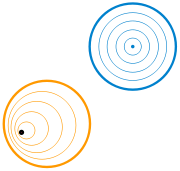The fundamental group of a plane minus a point (bold) consists of loops around the missing point. This group is isomorphic to the integers.

Examples and applications of groups abound. A starting point is the group Z of integers with addition as group operation, introduced above. If instead of addition multiplication is considered, one obtains multiplicative groups. These groups are predecessors of important constructions in abstract algebra.

Groups are also applied in many other mathematical areas. Mathematical objects are often examined by associating groups to them and studying the properties of the corresponding groups. For example, Henri Poincaré founded what is now called algebraic topology by introducing the fundamental group. By means of this connection, topological properties such as proximity and continuity translate into properties of groups.i[] For example, elements of the fundamental group are represented by loops. The second image at the right shows some loops in a plane minus a point. The blue loop is considered null-homotopic (and thus irrelevant), because it can be continuously shrunk to a point. The presence of the hole prevents the orange loop from being shrunk to a point. The fundamental group of the plane with a point deleted turns out to be infinite cyclic, generated by the orange loop (or any other loop winding once around the hole). This way, the fundamental group detects the hole.

In more recent applications, the influence has also been reversed to motivate geometric constructions by a group-theoretical background.j[] In a similar vein, geometric group theory employs geometric concepts, for example in the study of hyperbolic groups. Further branches crucially applying groups include algebraic geometry and number theory.

In addition to the above theoretical applications, many practical applications of groups exist. Cryptography relies on the combination of the abstract group theory approach together with algorithmical knowledge obtained in computational group theory, in particular when implemented for finite groups. Applications of group theory are not restricted to mathematics; sciences such as physics, chemistry and computer science benefit from the concept.

### Numbers

Many number systems, such as the integers and the rationals enjoy a naturally given group structure. In some cases, such as with the rationals, both addition and multiplication operations give rise to group structures. Such number systems are predecessors to more general algebraic structures known as rings and fields. Further abstract algebraic concepts such as modules, vector spaces and algebras also form groups.

#### Integers

The group of integers Z under addition, denoted (Z, +), has been described above. The integers, with the operation of multiplication instead of addition, (Z, ·) do not form a group. The closure, associativity and identity axioms are satisfied, but inverses do not exist: for example, a = 2 is an integer, but the only solution to the equation a · b = 1 in this case is b = 1/2, which is a rational number, but not an integer. Hence not every element of Z has a (multiplicative) inverse.k[]

#### Rationals

The desire for the existence of multiplicative inverses suggests considering fractionsFractions of integers (with b nonzero) are known as rational numbers.l[] The set of all such fractions is commonly denoted Q. There is still a minor obstacle for (Q, ·), the rationals with multiplication, being a group: because the rational number 0 does not have a multiplicative inverse (i.e., there is no x such that x · 0 = 1), (Q, ·) is still not a group.

However, the set of all nonzero rational numbers Q ∖ {0} = {qQ | q ≠ 0} does form an abelian group under multiplication, denoted (Q ∖ {0}, ·).m[] Associativity and identity element axioms follow from the properties of integers. The closure requirement still holds true after removing zero, because the product of two nonzero rationals is never zero. Finally, the inverse of a/b is b/a, therefore the axiom of the inverse element is satisfied.

The rational numbers (including 0) also form a group under addition. Intertwining addition and multiplication operations yields more complicated structures called rings and—if division is possible, such as in Q—fields, which occupy a central position in abstract algebra. Group theoretic arguments therefore underlie parts of the theory of those entities.n[]

### Modular arithmetic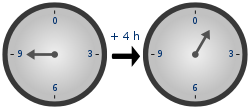The hours on a clock form a group that uses addition modulo 12. Here 9 + 4 = 1.

In modular arithmetic, two integers are added and then the sum is divided by a positive integer called the modulus. The result of modular addition is the remainder of that division. For any modulus, n, the set of integers from 0 to n − 1 forms a group under modular addition: the inverse of any element a is na, and 0 is the identity element. This is familiar from the addition of hours on the face of a clock: if the hour hand is on 9 and is advanced 4 hours, it ends up on 1, as shown at the right. This is expressed by saying that 9 + 4 equals 1 "modulo 12" or, in symbols,

9 + 4 ≡ 1 modulo 12.

The group of integers modulo n is written Zn or Z/nZ.

For any prime number p, there is also the multiplicative group of integers modulo p. Its elements are the integers 1 to p − 1. The group operation is multiplication modulo p. That is, the usual product is divided by p and the remainder of this division is the result of modular multiplication. For example, if p = 5, there are four group elements 1, 2, 3, 4. In this group, 4 · 4 = 1, because the usual product 16 is equivalent to 1, which divided by 5 yields a remainder of 1. for 5 divides 16 − 1 = 15, denoted

16 ≡ 1 (mod 5).

The primality of p ensures that the product of two integers neither of which is divisible by p is not divisible by p either, hence the indicated set of classes is closed under multiplication.o[] The identity element is 1, as usual for a multiplicative group, and the associativity follows from the corresponding property of integers. Finally, the inverse element axiom requires that given an integer a not divisible by p, there exists an integer b such that

a · b  1 (mod p), i.e. p divides the difference a · b − 1.

The inverse b can be found by using Bézout's identity and the fact that the greatest common divisor gcd(a, p) equals 1. In the case p = 5 above, the inverse of 4 is 4, and the inverse of 3 is 2, as 3 · 2 = 6 ≡ 1 (mod 5). Hence all group axioms are fulfilled. Actually, this example is similar to (Q ∖ {0}, ·) above: it consists of exactly those elements in Z/pZ that have a multiplicative inverse. These groups are denoted Fp×. They are crucial to public-key cryptography.p[]

### Cyclic groups

Main article: Cyclic group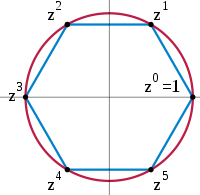The 6th complex roots of unity form a cyclic group. z is a primitive element, but z2 is not, because the odd powers of z are not a power of z2.

A cyclic group is a group all of whose elements are powers of a particular element a. In multiplicative notation, the elements of the group are:

..., a−3, a−2, a−1, a0 = e, a, a2, a3, ...,

where a2 means aa, and a−3 stands for a−1a−1a−1 = (aaa)−1 etc.h[] Such an element a is called a generator or a primitive element of the group. In additive notation, the requirement for an element to be primitive is that each element of the group can be written as

..., −aa, −a, 0, a, a+a, ...

In the groups Z/nZ introduced above, the element 1 is primitive, so these groups are cyclic. Indeed, each element is expressible as a sum all of whose terms are 1. Any cyclic group with n elements is isomorphic to this group. A second example for cyclic groups is the group of n-th complex roots of unity, given by complex numbers z satisfying zn = 1. These numbers can be visualized as the vertices on a regular n-gon, as shown in blue at the right for n = 6. The group operation is multiplication of complex numbers. In the picture, multiplying with z corresponds to a counter-clockwise rotation by 60°. Using some field theory, the group Fp× can be shown to be cyclic: for example, if p = 5, 3 is a generator since 31 = 3, 32 = 9 ≡ 4, 33 ≡ 2, and 34 ≡ 1.

Some cyclic groups have an infinite number of elements. In these groups, for every non-zero element a, all the powers of a are distinct; despite the name "cyclic group", the powers of the elements do not cycle. An infinite cyclic group is isomorphic to (Z, +), the group of integers under addition introduced above. As these two prototypes are both abelian, so is any cyclic group.

The study of finitely generated abelian groups is quite mature, including the fundamental theorem of finitely generated abelian groups; and reflecting this state of affairs, many group-related notions, such as center and commutator, describe the extent to which a given group is not abelian.

### Symmetry groups

Main article: Symmetry group

Symmetry groups are groups consisting of symmetries of given mathematical objects—be they of geometric nature, such as the introductory symmetry group of the square, or of algebraic nature, such as polynomial equations and their solutions. Conceptually, group theory can be thought of as the study of symmetry.t[] Symmetries in mathematics greatly simplify the study of geometrical or analytical objects. A group is said to act on another mathematical object X if every group element performs some operation on X compatibly to the group law. In the rightmost example below, an element of order 7 of the (2,3,7) triangle group acts on the tiling by permuting the highlighted warped triangles (and the other ones, too). By a group action, the group pattern is connected to the structure of the object being acted on.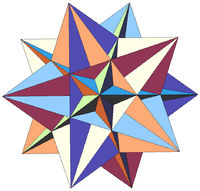Rotations and reflections form the symmetry group of a great icosahedron.

In chemical fields, such as crystallography, space groups and point groups describe molecular symmetries and crystal symmetries. These symmetries underlie the chemical and physical behavior of these systems, and group theory enables simplification of quantum mechanical analysis of these properties. For example, group theory is used to show that optical transitions between certain quantum levels cannot occur simply because of the symmetry of the states involved.

Not only are groups useful to assess the implications of symmetries in molecules, but surprisingly they also predict that molecules sometimes can change symmetry. The Jahn-Teller effect is a distortion of a molecule of high symmetry when it adopts a particular ground state of lower symmetry from a set of possible ground states that are related to each other by the symmetry operations of the molecule.

Likewise, group theory helps predict the changes in physical properties that occur when a material undergoes a phase transition, for example, from a cubic to a tetrahedral crystalline form. An example is ferroelectric materials, where the change from a paraelectric to a ferroelectric state occurs at the Curie temperature and is related to a change from the high-symmetry paraelectric state to the lower symmetry ferroelectric state, accompanied by a so-called soft phonon mode, a vibrational lattice mode that goes to zero frequency at the transition.

Such spontaneous symmetry breaking has found further application in elementary particle physics, where its occurrence is related to the appearance of Goldstone bosons.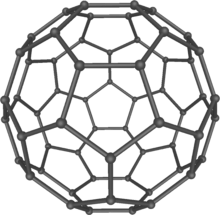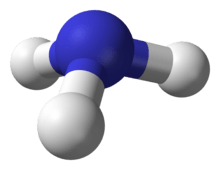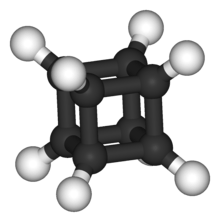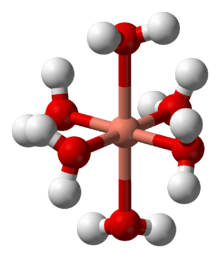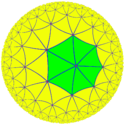Buckminsterfullerene displaysicosahedral symmetry, though the double bonds reduce this to pyritohedral symmetry. Ammonia, NH3. Its symmetry group is of order 6, generated by a 120° rotation and a reflection. Cubane C8H8 features octahedral symmetry. Hexaaquacopper(II) complex ion, [[copper|[Cu]](OH2)6]2+. Compared to a perfectly symmetrical shape, the molecule is vertically dilated by about 22% (Jahn-Teller effect). The (2,3,7) triangle group, a hyperbolic group, acts on this tiling of the hyperbolic plane.

Finite symmetry groups such as the Mathieu groups are used in coding theory, which is in turn applied in error correction of transmitted data, and in CD players. Another application is differential Galois theory, which characterizes functions having antiderivatives of a prescribed form, giving group-theoretic criteria for when solutions of certain differential equations are well-behaved.u[] Geometric properties that remain stable under group actions are investigated in (geometric) invariant theory.

### General linear group and representation theory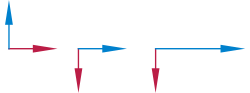Two vectors (the left illustration) multiplied by matrices (the middle and right illustrations). The middle illustration represents a clockwise rotation by 90°, while the right-most one stretches the x-coordinate by factor 2.

Matrix groups consist of matrices together with matrix multiplication. The general linear group GL(n, R) consists of all invertible n-by-n matrices with real entries. Its subgroups are referred to as matrix groups or linear groups. The dihedral group example mentioned above can be viewed as a (very small) matrix group. Another important matrix group is the special orthogonal group SO(n). It describes all possible rotations in n dimensions. Via Euler angles, rotation matrices are used in computer graphics.

Representation theory is both an application of the group concept and important for a deeper understanding of groups. It studies the group by its group actions on other spaces. A broad class of group representations are linear representations, i.e. the group is acting on a vector space, such as the three-dimensional Euclidean space R3. A representation of G on an n-dimensional real vector space is simply a group homomorphism

ρ: G → GL(n, R)

from the group to the general linear group. This way, the group operation, which may be abstractly given, translates to the multiplication of matrices making it accessible to explicit computations.w[]

Given a group action, this gives further means to study the object being acted on.x[] On the other hand, it also yields information about the group. Group representations are an organizing principle in the theory of finite groups, Lie groups, algebraic groups and topological groups, especially (locally) compact groups.

### Galois groups

Main article: Galois group

Galois groups were developed to help solve polynomial equations by capturing their symmetry features. For example, the solutions of the quadratic equation ax2 + bx + c = 0 are given byExchanging "+" and "−" in the expression, i.e. permuting the two solutions of the equation can be viewed as a (very simple) group operation. Similar formulae are known for cubic and quartic equations, but do not exist in general for degree 5 and higher. Abstract properties of Galois groups associated with polynomials (in particular their solvability) give a criterion for polynomials that have all their solutions expressible by radicals, i.e. solutions expressible using solely addition, multiplication, and roots similar to the formula above.

The problem can be dealt with by shifting to field theory and considering the splitting field of a polynomial. Modern Galois theory generalizes the above type of Galois groups to field extensions and establishes—via the fundamental theorem of Galois theory—a precise relationship between fields and groups, underlining once again the ubiquity of groups in mathematics.

## Finite groups

Main article: Finite group

A group is called finite if it has a finite number of elements. The number of elements is called the order of the group. An important class is the symmetric groups SN, the groups of permutations of N letters. For example, the symmetric group on 3 letters S3 is the group consisting of all possible orderings of the three letters ABC, i.e. contains the elements ABC, ACB, BAC, BCA, CAB, CBA, in total 6 (factorial of 3) elements. This class is fundamental insofar as any finite group can be expressed as a subgroup of a symmetric group SN for a suitable integer N, according to Cayley's theorem. Parallel to the group of symmetries of the square above, S3 can also be interpreted as the group of symmetries of an equilateral triangle.

The order of an element a in a group G is the least positive integer n such that a n = e, where a n representsi.e. application of the operation • to n copies of a. (If • represents multiplication, then an corresponds to the nth power of a.) In infinite groups, such an n may not exist, in which case the order of a is said to be infinity. The order of an element equals the order of the cyclic subgroup generated by this element.

More sophisticated counting techniques, for example counting cosets, yield more precise statements about finite groups: Lagrange's Theorem states that for a finite group G the order of any finite subgroup H divides the order of G. The Sylow theorems give a partial converse.

The dihedral group (discussed above) is a finite group of order 8. The order of r1 is 4, as is the order of the subgroup R it generates (see above). The order of the reflection elements fv etc. is 2. Both orders divide 8, as predicted by Lagrange's theorem. The groups Fp× above have order p − 1.

### Classification of finite simple groups

Mathematicians often strive for a complete classification (or list) of a mathematical notion. In the context of finite groups, this aim leads to difficult mathematics. According to Lagrange's theorem, finite groups of order p, a prime number, are necessarily cyclic (abelian) groups Zp. Groups of order p2 can also be shown to be abelian, a statement which does not generalize to order p3, as the non-abelian group D4 of order 8 = 23 above shows. Computer algebra systems can be used to list small groups, but there is no classification of all finite groups.q[] An intermediate step is the classification of finite simple groups.r[] A nontrivial group is called simple if its only normal subgroups are the trivial group and the group itself.s[] The Jordan–Hölder theorem exhibits finite simple groups as the building blocks for all finite groups. Listing all finite simple groups was a major achievement in contemporary group theory. 1998 Fields Medal winner Richard Borcherds succeeded in proving the monstrous moonshine conjectures, a surprising and deep relation between the largest finite simple sporadic group—the "monster group"—and certain modular functions, a piece of classical complex analysis, and string theory, a theory supposed to unify the description of many physical phenomena.

Many groups are simultaneously groups and examples of other mathematical structures. In the language of category theory, they are group objects in a category, meaning that they are objects (that is, examples of another mathematical structure) which come with transformations (called morphisms) that mimic the group axioms. For example, every group (as defined above) is also a set, so a group is a group object in the category of sets.

### Topological groups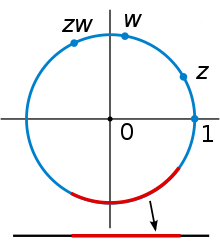The unit circle in the complex plane under complex multiplication is a Lie group and, therefore, a topological group. It is topological since complex multiplication and division are continuous. It is a manifold and thus a Lie group, because every small piece, such as the red arc in the figure, looks like a part of the real line (shown at the bottom).
Main article: Topological group

Some topological spaces may be endowed with a group law. In order for the group law and the topology to interweave well, the group operations must be continuous functions, that is, gh, and g−1 must not vary wildly if g and h vary only little. Such groups are called topological groups, and they are the group objects in the category of topological spaces. The most basic examples are the reals R under addition, (R ∖ {0}, ·), and similarly with any other topological field such as the complex numbers or p-adic numbers. All of these groups are locally compact, so they have Haar measures and can be studied via harmonic analysis. The former offer an abstract formalism of invariant integrals. Invariance means, in the case of real numbers for example:for any constant c. Matrix groups over these fields fall under this regime, as do adele rings and adelic algebraic groups, which are basic to number theory. Galois groups of infinite field extensions such as the absolute Galois group can also be equipped with a topology, the so-called Krull topology, which in turn is central to generalize the above sketched connection of fields and groups to infinite field extensions. An advanced generalization of this idea, adapted to the needs of algebraic geometry, is the étale fundamental group.

### Lie groups

Main article: Lie group

Lie groups (in honor of Sophus Lie) are groups which also have a manifold structure, i.e. they are spaces looking locally like some Euclidean space of the appropriate dimension. Again, the additional structure, here the manifold structure, has to be compatible, i.e. the maps corresponding to multiplication and the inverse have to be smooth.

A standard example is the general linear group introduced above: it is an open subset of the space of all n-by-n matrices, because it is given by the inequality

det (A) ≠ 0,

where A denotes an n-by-n matrix.

Lie groups are of fundamental importance in modern physics: Noether's theorem links continuous symmetries to conserved quantities. Rotation, as well as translations in space and time are basic symmetries of the laws of mechanics. They can, for instance, be used to construct simple models—imposing, say, axial symmetry on a situation will typically lead to significant simplification in the equations one needs to solve to provide a physical description.v[] Another example are the Lorentz transformations, which relate measurements of time and velocity of two observers in motion relative to each other. They can be deduced in a purely group-theoretical way, by expressing the transformations as a rotational symmetry of Minkowski space. The latter serves—in the absence of significant gravitation—as a model of space time in special relativity. The full symmetry group of Minkowski space, i.e. including translations, is known as the Poincaré group. By the above, it plays a pivotal role in special relativity and, by implication, for quantum field theories. Symmetries that vary with location are central to the modern description of physical interactions with the help of gauge theory.

## Generalizations

Group-like structures
Totalityα Associativity Identity Invertibility Commutativity
Semicategory Unneeded Required Unneeded Unneeded Unneeded
Category Unneeded Required Required Unneeded Unneeded
Groupoid Unneeded Required Required Required Unneeded
Magma Required Unneeded Unneeded Unneeded Unneeded
Quasigroup Required Unneeded Unneeded Required Unneeded
Loop Required Unneeded Required Required Unneeded
Semigroup Required Required Unneeded Unneeded Unneeded
Monoid Required Required Required Unneeded Unneeded
Group Required Required Required Required Unneeded
Abelian Group Required Required Required Required Required
Closure, which is used in many sources, is an equivalent axiom to totality, though defined differently.

In abstract algebra, more general structures are defined by relaxing some of the axioms defining a group. For example, if the requirement that every element has an inverse is eliminated, the resulting algebraic structure is called a monoid. The natural numbers N (including 0) under addition form a monoid, as do the nonzero integers under multiplication (Z ∖ {0}, ·), see above. There is a general method to formally add inverses to elements to any (abelian) monoid, much the same way as (Q ∖ {0}, ·) is derived from (Z ∖ {0}, ·), known as the Grothendieck group. Groupoids are similar to groups except that the composition ab need not be defined for all a and b. They arise in the study of more complicated forms of symmetry, often in topological and analytical structures, such as the fundamental groupoid or stacks. Finally, it is possible to generalize any of these concepts by replacing the binary operation with an arbitrary n-ary one (i.e. an operation taking n arguments). With the proper generalization of the group axioms this gives rise to an n-ary group. The table gives a list of several structures generalizing groups.

## Notes

^ a: Mathematical Reviews lists 3,224 research papers on group theory and its generalizations written in 2005.
^ aa: The classification was announced in 1983, but gaps were found in the proof. See classification of finite simple groups for further information.
^ b: The closure axiom is already implied by the condition that • be a binary operation. Some authors therefore omit this axiom. However, group constructions often start with an operation defined on a superset, so a closure step is common in proofs that a system is a group. Lang 2002
^ c: See, for example, the books of Lang (2002, 2005) and Herstein (1996, 1975).
^ d: However, a group is not determined by its lattice of subgroups. See Suzuki 1951.
^ e: The fact that the group operation extends this canonically is an instance of a universal property.
^ f: For example, if G is finite, then the size of any subgroup and any quotient group divides the size of G, according to Lagrange's theorem.
^ g: The word homomorphism derives from Greek ὁμός—the same and μορφή—structure.
^ h: The additive notation for elements of a cyclic group would be ta, t in Z.
^ i: See the Seifert–van Kampen theorem for an example.
^ j: An example is group cohomology of a group which equals the singular cohomology of its classifying space.
^ k: Elements which do have multiplicative inverses are called units, see Lang 2002,§II.1, p. 84.
^ l: The transition from the integers to the rationals by adding fractions is generalized by the quotient field.
^ m: The same is true for any field F instead of Q. See Lang 2005,§III.1, p. 86.
^ n: For example, a finite subgroup of the multiplicative group of a field is necessarily cyclic. See Lang 2002,Theorem IV.1.9. The notions of torsion of a module and simple algebras are other instances of this principle.
^ o: The stated property is a possible definition of prime numbers. See prime element.
^ p: For example, the Diffie-Hellman protocol uses the discrete logarithm.
^ q: The groups of order at most 2000 are known. Up to isomorphism, there are about 49 billion. See Besche, Eick & O'Brien 2001.
^ r: The gap between the classification of simple groups and the one of all groups lies in the extension problem, a problem too hard to be solved in general. See Aschbacher 2004,p. 737.
^ s: Equivalently, a nontrivial group is simple if its only quotient groups are the trivial group and the group itself. See Michler 2006, Carter 1989.
^ t: More rigorously, every group is the symmetry group of some graph; see Frucht's theorem, Frucht 1939.
^ u: More precisely, the monodromy action on the vector space of solutions of the differential equations is considered. See Kuga 1993,pp. 105–113.
^ v: See Schwarzschild metric for an example where symmetry greatly reduces the complexity of physical systems.
^ w: This was crucial to the classification of finite simple groups, for example. See Aschbacher 2004.
^ x: See, for example, Schur's Lemma for the impact of a group action on simple modules. A more involved example is the action of an absolute Galois group on étale cohomology.
^ y: Injective and surjective maps correspond to mono- and epimorphisms, respectively. They are interchanged when passing to the dual category.

## Citations

1. Herstein 1975,§2, p. 26
2. Hall 1967,§1.1, p. 1: "The idea of a group is one which pervades the whole of mathematics both pure and applied."
3. Lang 2005,App. 2, p. 360
4. Cook, Mariana R. (2009), Mathematicians: An Outer View of the Inner World, Princeton, N.J.: Princeton University Press, p. 24, ISBN 9780691139517
5. Herstein 1975,§2.1, p. 27
6. Herstein 1975,§2.6, p. 54
7. Wussing 2007
8. Kleiner 1986
9. Smith 1906
10. Galois 1908
11. Kleiner 1986,p. 202
12. Cayley 1889
13. Wussing 2007,§III.2
14. Lie 1973
15. Kleiner 1986,p. 204
16. Wussing 2007,§I.3.4
17. Jordan 1870
18. von Dyck 1882
19. Curtis 2003
20. Mackey 1976
21. Borel 2001
22. Aschbacher 2004
23. Ledermann 1953,§1.2, pp. 4–5
24. Ledermann 1973,§I.1, p. 3
25. Lang 2002,§I.2, p. 7
26. Lang 2005,§II.1, p. 17
27. Mac Lane 1998
28. Lang 2005,§II.3, p. 34
29. Lang 2005,§II.1, p. 19
30. Ledermann 1973,§II.12, p. 39
31. Lang 2005,§II.4, p. 41
32. Lang 2002,§I.2, p. 12
33. Lang 2005,§II.4, p. 45
34. Lang 2002,§I.2, p. 9
35. Hatcher 2002,Chapter I, p. 30
36. Coornaert, Delzant & Papadopoulos 1990
37. for example, class groups and Picard groups; see Neukirch 1999, in particular §§I.12 and I.13
38. Seress 1997
39. Lang 2005,Chapter VII
40. Rosen 2000,p. 54 (Theorem 2.1)
41. Lang 2005,§VIII.1, p. 292
42. Lang 2005,§II.1, p. 22
43. Lang 2005,§II.2, p. 26
44. Lang 2005,§II.1, p. 22 (example 11)
45. Lang 2002,§I.5, p. 26, 29
46. Weyl 1952
48. Bersuker, Isaac (2006), The Jahn-Teller Effect, Cambridge University Press, p. 2, ISBN 0-521-82212-2
49. Jahn & Teller 1937
50. Dove, Martin T (2003), Structure and Dynamics: an atomic view of materials, Oxford University Press, p. 265, ISBN 0-19-850678-3
51. Welsh 1989
52. Mumford, Fogarty & Kirwan 1994
53. Lay 2003
54. Kuipers 1999
55. Fulton & Harris 1991
56. Serre 1977
57. Rudin 1990
58. Robinson 1996,p. viii
59. Artin 1998
60. Lang 2002,Chapter VI (see in particular p. 273 for concrete examples)
61. Lang 2002,p. 292 (Theorem VI.7.2)
62. Kurzweil & Stellmacher 2004
63. Artin 1991,Theorem 6.1.14. See also Lang 2002,p. 77 for similar results.
64. Lang 2002,§I. 3, p. 22
65. Ronan 2007
66. Husain 1966
67. Neukirch 1999
68. Shatz 1972
69. Milne 1980
70. Warner 1983
71. Borel 1991
72. Goldstein 1980
73. Weinberg 1972
74. Naber 2003
75. Becchi 1997
76. Denecke & Wismath 2002
77. Romanowska & Smith 2002
78. Dudek 2001

## References

### General references

• Artin, Michael (1991), Algebra, Prentice Hall, ISBN 978-0-89871-510-1, Chapter 2 contains an undergraduate-level exposition of the notions covered in this article.
• Devlin, Keith (2000), The Language of Mathematics: Making the Invisible Visible, Owl Books, ISBN 978-0-8050-7254-9, Chapter 5 provides a layman-accessible explanation of groups.
• Fulton, William; Harris, Joe (1991). Representation theory. A first course. Graduate Texts in Mathematics, Readings in Mathematics. 129. New York: Springer-Verlag. ISBN 978-0-387-97495-8. MR 1153249, ISBN 978-0-387-97527-6..
• Hall, G. G. (1967), Applied group theory, American Elsevier Publishing Co., Inc., New York, MR 0219593, an elementary introduction.
• Herstein, Israel Nathan (1996), Abstract algebra (3rd ed.), Upper Saddle River, NJ: Prentice Hall Inc., ISBN 978-0-13-374562-7, MR 1375019.
• Herstein, Israel Nathan (1975), Topics in algebra (2nd ed.), Lexington, Mass.: Xerox College Publishing, MR 0356988.
• Lang, Serge (2002), Algebra, Graduate Texts in Mathematics, 211 (Revised third ed.), New York: Springer-Verlag, ISBN 978-0-387-95385-4, MR 1878556
• Lang, Serge (2005), Undergraduate Algebra (3rd ed.), Berlin, New York: Springer-Verlag, ISBN 978-0-387-22025-3.
• Ledermann, Walter (1953), Introduction to the theory of finite groups, Oliver and Boyd, Edinburgh and London, MR 0054593.
• Ledermann, Walter (1973), Introduction to group theory, New York: Barnes and Noble, OCLC 795613.
• Robinson, Derek John Scott (1996), A course in the theory of groups, Berlin, New York: Springer-Verlag, ISBN 978-0-387-94461-6.